Data Analysis with R

3 - Data structures and basic calculations

Postdoctoral Researcher

Some recap on data structures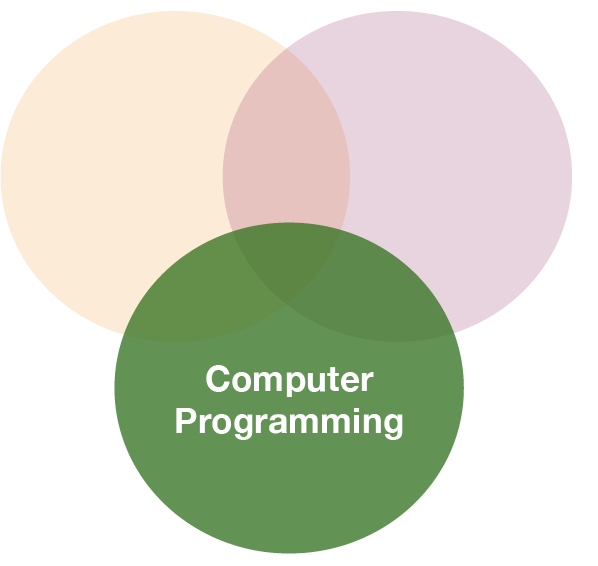Data structures

Five data types most often used in data analysis:

Dimensions Homogeneous Heterogeneous
1d Atomic vector List
2d Matrix Data frame
nd Array

ListsLists

• are different from atomic vectors because their elements can be of any type, including lists
• you construct lists by using list() instead of c():
x <- list(1:3, "a", c(TRUE, FALSE, TRUE), c(2.3, 5.9))
str(x)
## List of 4
##  $: int [1:3] 1 2 3 ##$ : chr "a"
##  $: logi [1:3] TRUE FALSE TRUE ##$ : num [1:2] 2.3 5.9

Lists are vectors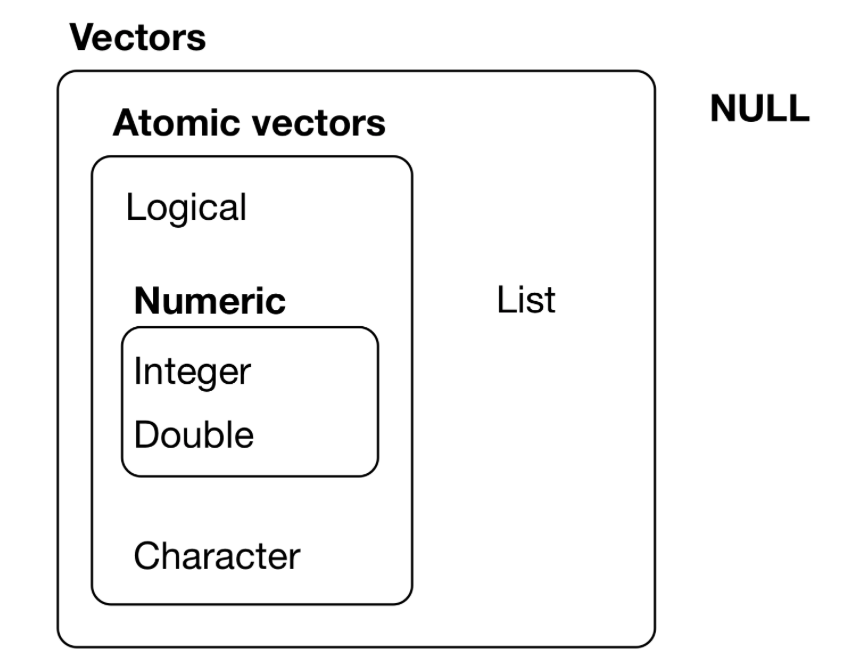NULL is often used to represent the absence of a vector (as opposed to NA which is used to represent the absence of a value in a vector). NULL typically behaves like a vector of length 0.

Why is a list considered a vector?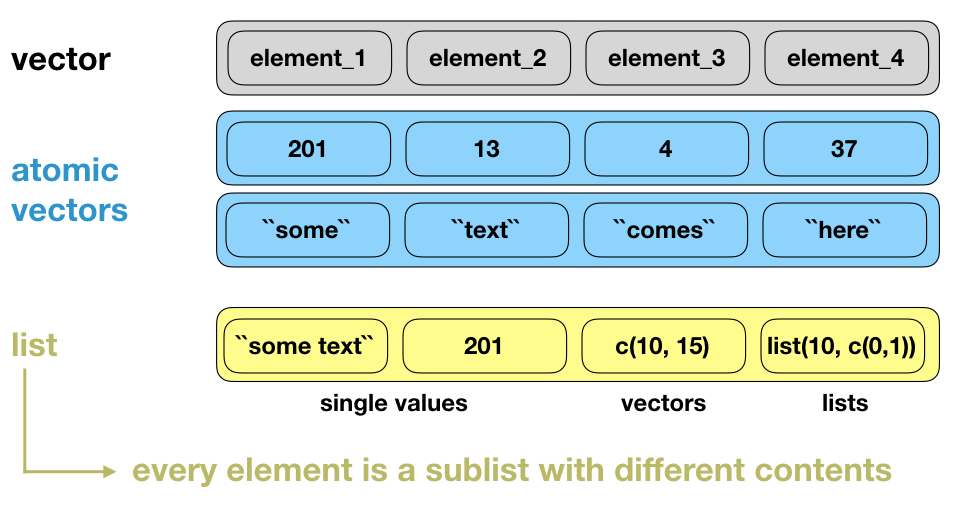Lists (cont)

• are sometimes called recursive vectors, because a list can contain other lists.
x <- list(list(list(list())))
str(x)
## List of 1
##  $:List of 1 ## ..$ :List of 1
##   .. ..$: list() Visualization of the following lists x1 <- list(c(1, 2), c(3, 4)) x2 <- list(list(1, 2), list(3, 4)) x3 <- list(1, list(2, list(3)))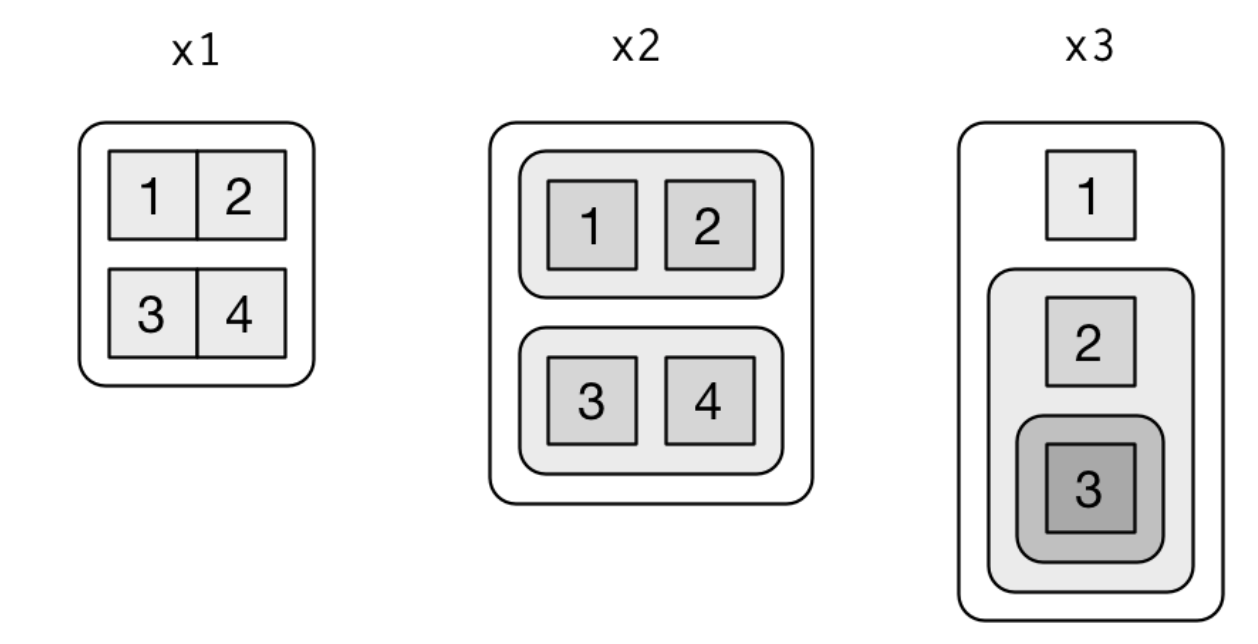source: R for Data Science by Wickam & Grolemund, 2017 (licensed under CC-BY-NC-ND 3.0 US) Lists (cont) • typeof() a list is a list • you can test for a list with is.list() and • coerce to a list with as.list() • you can turn a list into an atomic vector with unlist(). • if the elements of a list have different types, unlist() uses the same coercion rules as c(). • lists are used to build up many of the more complicated data structures in R. Structure of lists A very useful tool for working with lists is str() because it focuses on the structure, not the content. x <- list(1, 2, 3) str(x) ## List of 3 ##$ : num 1
##  $: num 2 ##$ : num 3

Structure of lists

A very useful tool for working with lists is str() because it focuses on the structure, not the content.

x <- list(1, 2, 3)
str(x)
## List of 3
##  $: num 1 ##$ : num 2
##  $: num 3 x_named <- list(a = 1, b = 2, c = 3) str(x_named) ## List of 3 ##$ a: num 1
##  $b: num 2 ##$ c: num 3

Subsetting

Three ways to subset a list:

1. [ extracts a sublist.
2. [[ extracts a single component from a list.
3. $is a shorthand for extracting named elements of a list. Subsetting (cont) I will demonstrate each way using the following list: a <- list(a = 1:3, b = "a string", c = pi, list(-1, -5)) str(a) ## List of 4 ##$ a: int [1:3] 1 2 3
##  $b: chr "a string" ##$ c: num 3.14
##  $:List of 2 ## ..$ : num -1
##   ..$: num -5 Subsetting: '[ ]' 1.[ extracts a sublist. The result will always be a list (it keeps the original list 'container' and removes all elements not selected). Like with vectors, you can subset with a logical, integer, or character vector. str(a[1:2]) ## List of 2 ##$ a: int [1:3] 1 2 3
##  $b: chr "a string" str(a) ## List of 1 ##$ :List of 2
##   ..$: num -1 ## ..$ : num -5

Subsetting: '[ ]'

1.[ extracts a sublist. The result will always be a list (it keeps the original list 'container' and removes all elements not selected). Like with vectors, you can subset with a logical, integer, or character vector.

str(a[1:2])
## List of 2
##  $a: int [1:3] 1 2 3 ##$ b: chr "a string"
str(a)
## List of 1
##  $:List of 2 ## ..$ : num -1
##  $: num -5 a[] ## [] ##  -1 ## ## [] ##  -5 Subsetting: '$'

3.$is a shorthand for extracting named elements of a list. It works similarly to [[ except that you don’t need to use quotes. a$a
##  1 2 3
# same as
a[["a"]]
##  1 2 3

Visualize the following lists as nested sets

1. list(a, b, list(c, d), list(e, f))

2. list(list(list(list(list(list(a))))))

Quiz 1: Subsetting lists

The following list has been created:

list_example <- list(one = 1:10, two = letters,
three = list(abc = c(132, 876, 42), xyz = c(T,F,F,T,F,T)), four = NULL)

What does the following return? list_example[1:2]

1. a vector with the first 2 elements of each list
2. a list of all sublists, each containing only the first 2 elements of the original sublists
3. a list containing only sublist "one" and "two"
4. NA

[ extracts always sublists and returns a list. In this example, you extract the first and second sublists, which are the numeric vector "one" and the character vector "two".

Quiz 2: Subsetting lists

The following list has been created:

list_example <- list(one = 1:10, two = letters,
three = list(abc = c(132, 876, 42), xyz = c(T,F,F,T,F,T)), four = NULL)

What does the following return? list_example["four"]

1. NULL
2. error message
3. a list containing NULL
4. a vector with NULL elements

[ extracts always sublists and returns a list. In this example, you extract the sublist that is called "four", which is the fourth sublist containing NULL elements.

Quiz 3: Subsetting lists

The following list has been created:

list_example <- list(one = 1:10, two = letters,
three = list(abc = c(132, 876, 42), xyz = c(T,F,F,T,F,T)), four = NULL)

What does the following return? list_example[]

1. a list containing "a"
2. a list containing 1
3. a vector containing "b"
4. a vector containing 2

[[ extracts always single components from a list. In this case, it extracts the first sublist, which is a vector, and from there the 2nd element, which is a 2. So the returned object is also a vector containing only the number 2.

Quiz 4: Subsetting lists

The following list has been created:

list_example <- list(one = 1:10, two = letters,
three = list(abc = c(132, 876, 42), xyz = c(T,F,F,T,F,T)), four = NULL)

What does the following return? list_example[]

1. NA
2. a list containing FALSE
3. the logical vector 'xyz'
4. a vector containing "c"
5. error message

This is a bit trickier! The code snippet tries to subset the following: return a list that extracts from 'list_example' the 3rd sublist and from this list take sublist 2. BUT: that list has only 1 element, which is the list 'three' (containing vectors 'abc' and 'xyz'); a sublist 2 doesn't exist. However, the following would have worked: list_example[2:3][] since now the extracted list contains 2 sublists, "two" and "three", from which it can subset list "three".

Quiz 5: Subsetting lists

What is equivalent to the following code (multiple answers correct)? And which of the options below returns a suprising value?

list_example[["three"]][c("abc", "xyz")]

1. list_example[][1:2]
2. list_example[][[1:2]]
3. list_example[][c("abc", "xyz")]
4. list_example$three[1:2] 5. list_example$three[c("abc", "xyz")]

Option 2 subsets something else, which is not so intuitive: it extracts also the 3rd sublist "three", but from there it subsets the 2nd element of the 1st sublist (the vector "abc"). DO NOT use this notation for extracting the number '876', use instead: list_example[][] (element 2 in sublist 1 within sublist 3)!!!

Quiz 6 - Challenge: Subsetting lists

Create a new vector that contains the 4th element of sublist "one" and element 1 and 3 from sublist "abc" within "three" in 'list_example'.

1. What is the sum of this vector?

Within the vector you extract first the value from sublist "one" and then values from sublist "abc" within sublist "three" (both seperated by a comma).

To get the correct answer you could subset for instance like this:
sum( c(list_example[], list_example[][][c(1,3)]) )
or using the $sign: sum( c(list_example$one, list_example$three$abc[c(1,3)]) )

1. 176

Quiz 7 - Challenge: Subsetting lists

Execute the following R command in your console

lm_list <- lm(Sepal.Length ~ Sepal.Width, data = iris)

and look at the structure of the list you created with

str(lm_list, max.level = 1) # max.level=1 shows only the first level
# of the hierarchy (and not all sub/sub/..lists))
1. What is the last value of the 'residuals'?

Look at the names of the sublists in lm_list. Is there anything that sounds like what we are looking for? If you found it check the number of elements it contains (we want the last one!).

##  $y: Factor w/ 3 levels "a","b","c": 1 2 3 Generating data frames You create a data frame using data.frame() (note the point inbetween both words!), which takes named vectors as input: df <- data.frame(x = 1:3, y = c("a", "b", "c")) str(df) ## 'data.frame': 3 obs. of 2 variables: ##$ x: int  1 2 3
##  $y: Factor w/ 3 levels "a","b","c": 1 2 3 • Beware of data.frame()s default behaviour, which turns strings into factors. Use stringsAsFactors = FALSE to suppress this behaviour: df <- data.frame(x = 1:3, y = c("a", "b", "c"), stringsAsFactors = FALSE) str(df) ## 'data.frame': 3 obs. of 2 variables: ##$ x: int  1 2 3
##  1 2 3

Quiz 15: iris dataset - subsetting

1. Calculate the sum of all observations in the dataset using the function sum()

The easiest is to subset matrix-like within the sum function: sum( iris[, 1:4] )

1. 2078.7

The workspace or the global environmentWhen you create R objects, you'll see them appear in your environment pane under Global Environment:

x <- 1:10
y <- 1:10
z <- cbind(x,y)  # matrix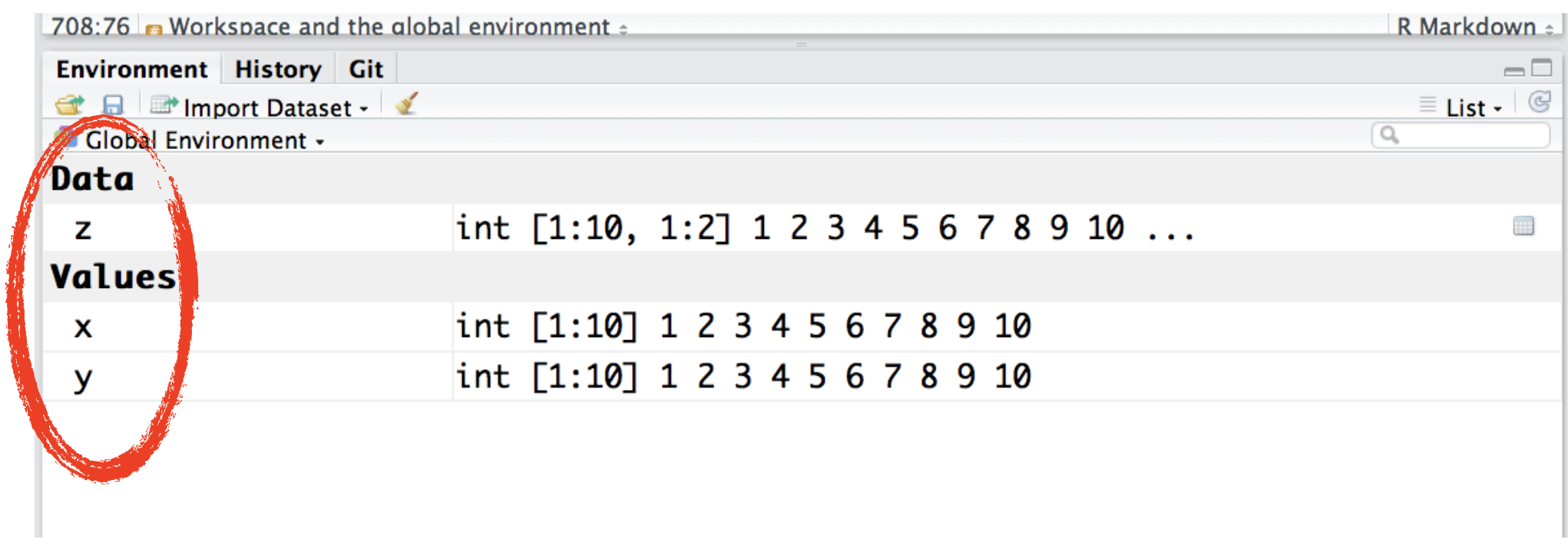The global environment, more often known as the user's workspace, is the first item on the search path. When a user starts a new session in R, the R system creates a new environment for objects created during that session.

You can list all objects in the workspace using the function ls():

x <- 1:10
y <- 1:10
z <- cbind(x,y)  # matrix
ls()
##  "x" "y" "z"

Remove objects from workspace

You can remove an object with rm():

x <- 4
x
rm(x)

Remove objects from workspace

You can remove an object with rm():

x <- 4
x
rm(x)

Or remove all objects in one go: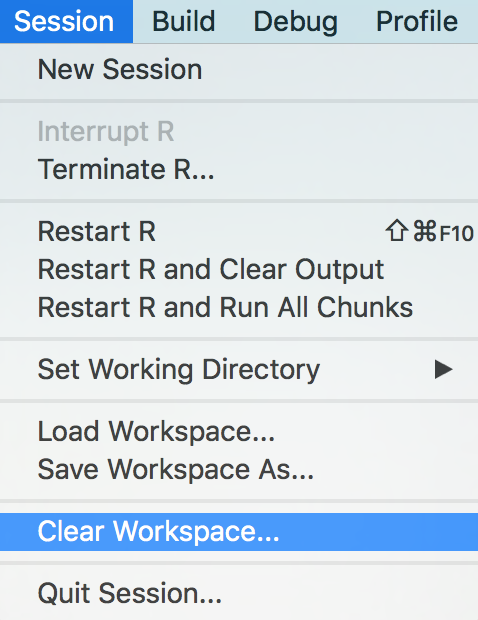Overview of functions you learned today

str(), [, [[, \$,

list, is.list(), as.list(), unlist(),

matrix(), cbind(), nrow(), ncol(), dim, rownames(), colnames(), dimnames(),

data.frame(), data.frame(stringsAsFactors = FALSE)

How do you feel now.....?

Totally confused?Again, try out the online tutorial at Data Camp.

And go over this lecture again and do the quizzes.

Totally bored?Then try out the following: Calculate for the iris data set

• the mean sepal and petal length per species, and
• the minimum petal width for the species "setose".
• Which species has the longest sepal width?

Totally content?

Then go grab a coffee, lean back and enjoy the rest of the day...!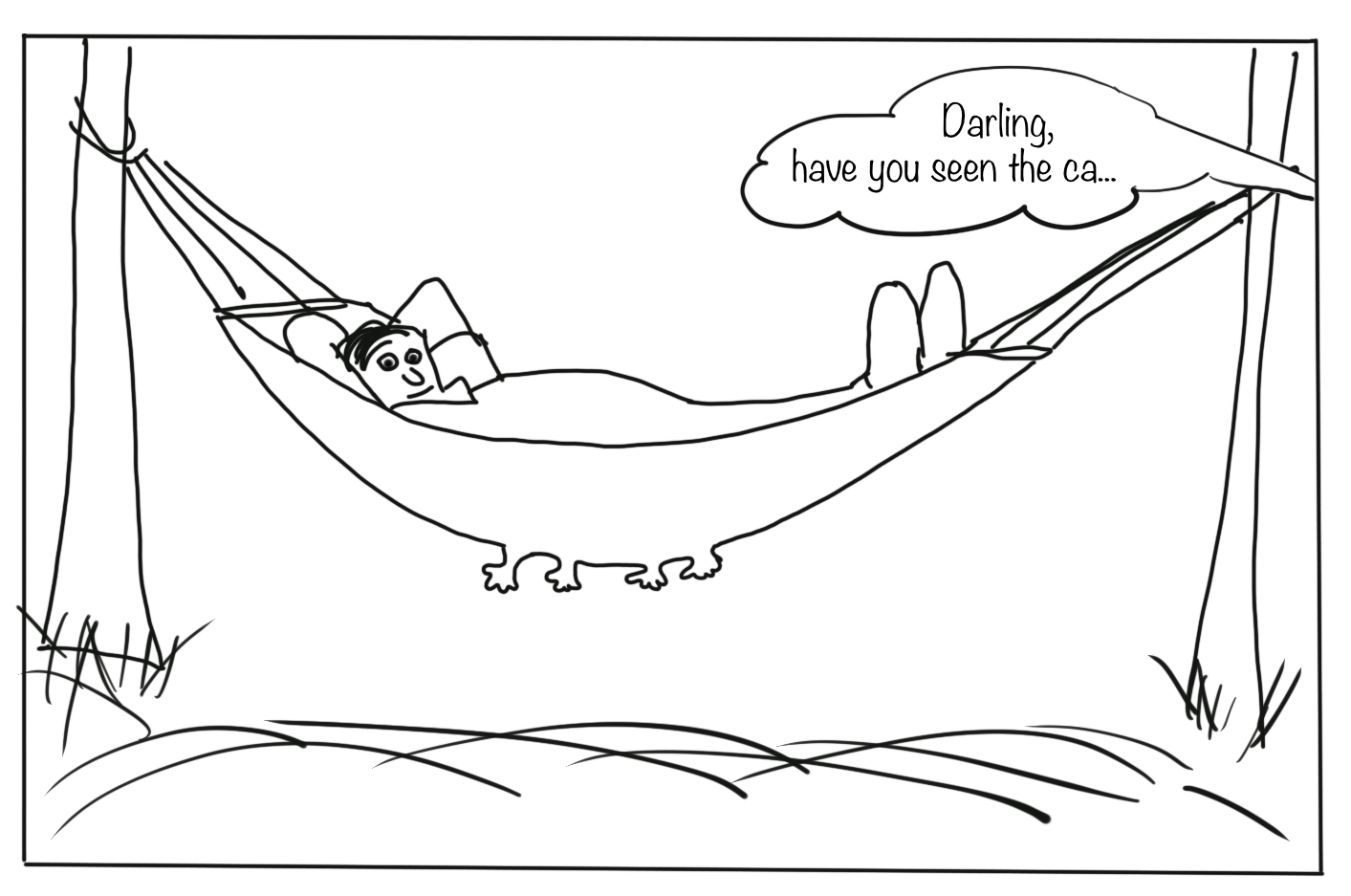Thank You